Win up to 100% scholarship on Aakash BYJU'S JEE/NEET courses with ABNAT Win up to 100% scholarship on Aakash BYJU'S JEE/NEET courses with ABNAT

# JEE Main 2020 (Shift 2-3rd Sept) Physics Paper With Solutions

Practising JEE Main 2020 (Shift 2-3rd Sept) questions help the students to get an idea about the type of questions that can appear in the upcoming JEE exams. Solving previous years’ question papers always be helpful to increase problem-solving speed and to understand the pattern of the question paper. Click on the link below to download the Physics 2020 Shift 2 paper.

### JEE Main 2020 (Shift 2-3rd Sept) Physics Paper With Solutions

September 3 Shift 2 – Physics

1. A block of mass m attached to a massless spring is performing oscillatory motion of amplitude ‘A’ on a frictionless horizontal plane. If half of the mass of the block breaks off when it is passing through its equilibrium point, the amplitude of oscillation for the remaining system become fA. The value of f is:

1) 1/√2
2) 1/2
3) 1
4)√ 2

V1 = Vmax = Aω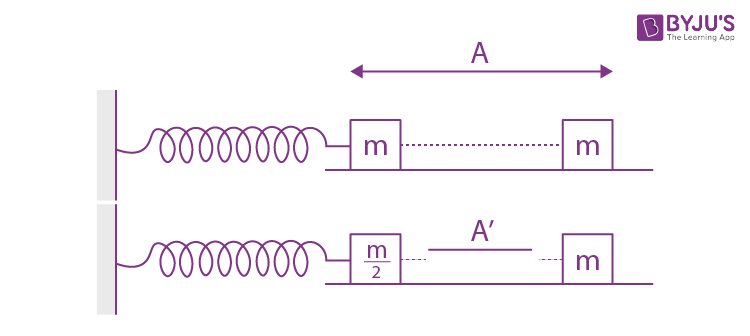V2 = Vmax = A’ω’

Aω = A’ω’

A(√k/√m) = A’(√2k/√m)

A’ = A/√2

2. The mass density of a planet of radius R varies with the distance r from its centre as ρ(r) = ρ 0(1-(r2/R2)). Then the gravitational field is maximum at:

1) r =1/√3R
2) r = (√3/√4)R
3) r = R
4) r = (√5/√9R)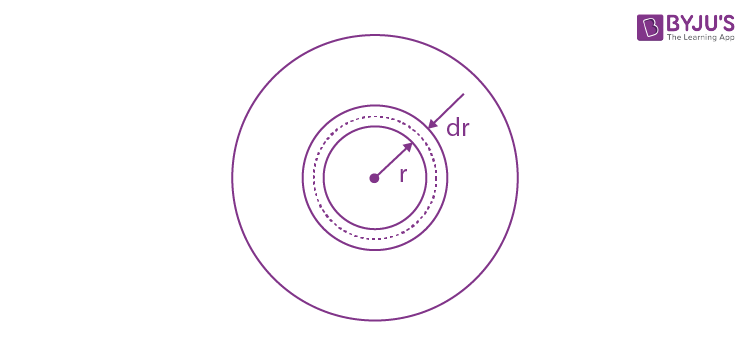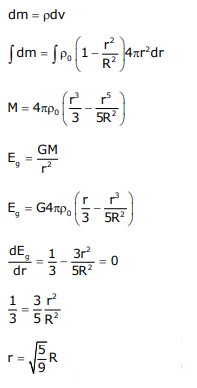3. Two sources of light emit X-rays of wavelength 1 nm and visible light of wavelength 500 nm, respectively. Both the sources emit light of the same power 200 W. The ratio of the number density of photons of X-rays to the number density of photons of the visible light of the given wavelengths is:

1) 1/500
2) 1/250
3) 500
4) 250

$$\begin{array}{l}\frac{1}{2}mv^{2} = p \times t\end{array}$$
$$\begin{array}{l}v = \alpha \sqrt{t}\end{array}$$
$$\begin{array}{l}\frac{ds}{dt} = \alpha \sqrt{t}\end{array}$$
$$\begin{array}{l}S\: \alpha \: t^{\frac{3}{2}}\end{array}$$

4. If a semiconductor photodiode can detect a photon with a maximum wavelength of 400nm, then its band gap energy is : Planck’s constant h = 6.63 × 10–34 J.s. Speed of light c = 3 × 108 m/s

1) 1.5 eV
2) 2.0 eV
3) 3.1 eV
4) 1.1 eV

E = (hc/λ)

E = (6.63 x 10-34 x 3 x 108)/(400 x 10-9)

E = (1240/400) eV

E = 3.1 eV

5. Amount of solar energy received on the earth’s surface per unit area per unit time is defined a solar constant. Dimension of solar constant is:

1) ML0T–3
2) MLT–2
3) M2L0T–1
4) ML2T–2

E = (Q/At)

E = ML2T-2/L2T

E = MT-3

6. A particle is moving unidirectionally on a horizontal plane under the action of a constant power supplying energy source. The displacement (s) – time (t) graph that describes the motion of the particle is (graphs are drawn schematically and are not to scale: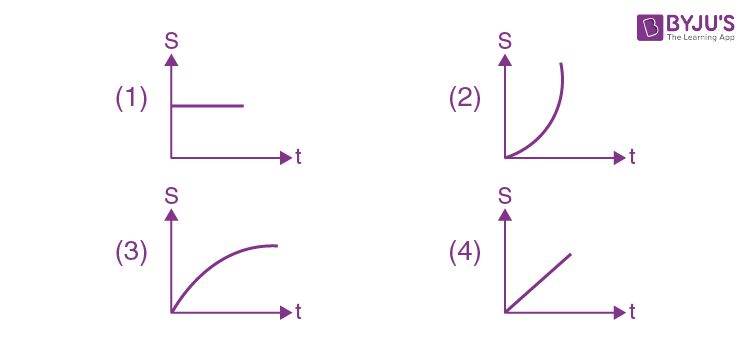P = FV

P = m (dv/ dt) v

vdv =(P/m) dt

V2 =k’tV = K’’√t

S ∝ t3/2

7. Which of the following will NOT be observed when a multimeter (operating in resistance measuring mode) probes connected across a component, are just reversed?

1) Multimeter shows NO deflection in both cases i.e. before and after reversing the probes if the chosen component is metal wire.
2) Multimeter shows a deflection, accompanied by a splash of light out of connected component in one direction and NO deflection on reversing the probes if the chosen component is LED.
3) Multimeter shows an equal deflection in both cases i.e. before and after reversing the probes if the chosen component is resistor.
4) Multimeter shows NO deflection in both cases i.e. before and after reversing the probes if the chosen component is capacitor.

By Theory

8. A uniform rod of length ‘l’ is pivoted at one of its ends on a vertical shaft of negligible radius. When the shaft rotates at angular speed ω the rod makes an angle θ with it (see figure). To find θ equate the rate of change of angular momentum (direction going into the paper) (ml2/12) ω2sinθcosθ about the centre of mass (CM) to the torque provided by the horizontal and vertical forces FH and Fv about the CM. The value of θ is then such that: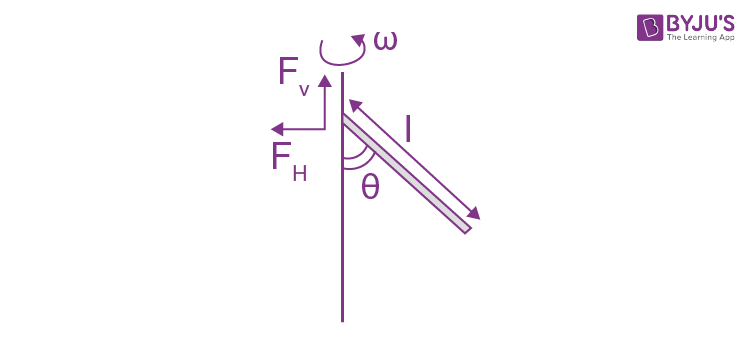1) cos θ = 2g/3l ω2
2) cos θ = 3g/2l ω2
3) cos θ = g/2l ω2
4) cos θ = g/I ω2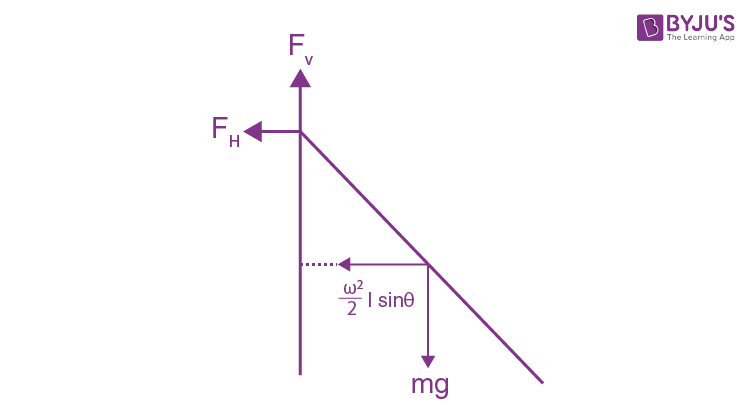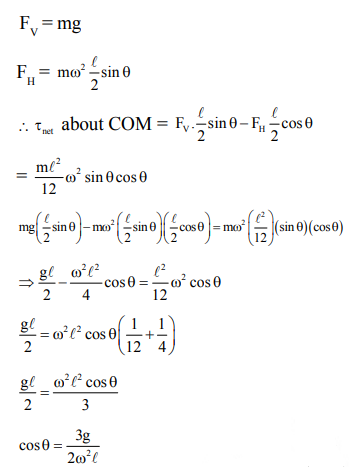9. Two resistors 400 Ω and 800 Ω are connected in series across a 6 V battery. The potential difference measured by a voltmeter of 10 k Ω across 400 Ω resistors is close to:

1) 2.05 V
2) 2 V
3) 1.95 V
4) 1.8 V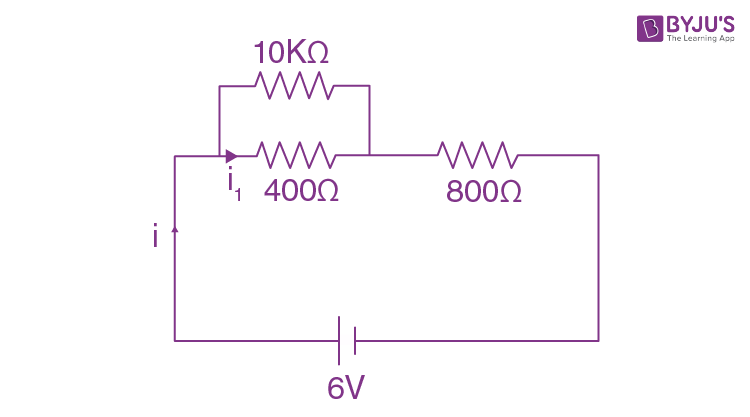$$\begin{array}{l}i=\frac{6}{800+\frac{400\times 10000}{400+10000}}\end{array}$$

I = 6/1184.61 = 0.00506

Vv = 6-800 x 0.00506 = 6 – 4.05 = 1.95

10. A block of mass 1.9 kg is at rest at the edge of a table, of height 1 m. A bullet of mass 0.1 kg collides with the block and sticks to it. If the velocity of the bullet is 20 m/s in the horizontal direction just before the collision then the kinetic energy just before the combined system strikes the floor, is [Take g = 10 m/s2 . Assume there is no rotational motion and loss of energy after the collision is negligible.]

1) 23 J
2) 21 J
3) 20 J
4) 19 J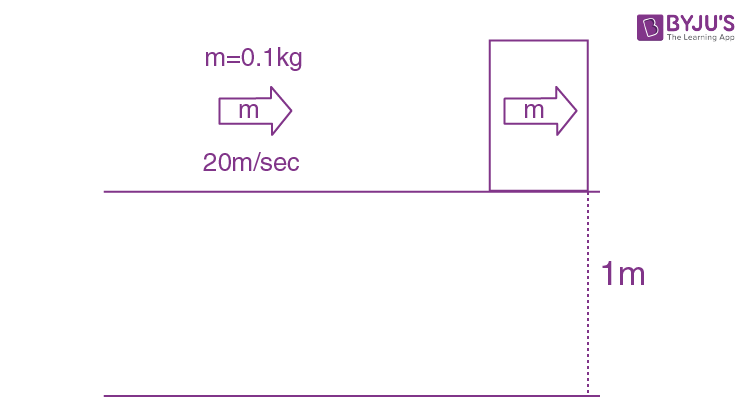Applying law of conservation of linear momentum

0.1×20 = (1.9+0.1)V

2 = 2 V

V = 1 m/sec

KE= (1/2) mv2 = (1/2) 2(1)2 = 1J

TE = KE + mgh = 1+2×10×1 = 21J

11. A metallic sphere cools from 50°C to 40°C in 300 s. If atmospheric temperature around is 20°C, then the sphere’s temperature after the next 5 minutes will be close to :

1) 35°C
2) 31°C
3) 33°C
4) 28°C

$$\begin{array}{l}\frac{\Delta T}{\Delta t}=k\left [ \frac{T_{f}+T_{i}}{2}-T_{0} \right ]\end{array}$$
$$\begin{array}{l}\frac{50-40}{300}=k\left [ \frac{90}{2}-20 \right ]\end{array}$$
$$\begin{array}{l}\frac{40-T}{300}=k\left [ \frac{40+T}{2}-20 \right ]\end{array}$$
$$\begin{array}{l}\frac{10}{40-T}=\left [ \frac{25\times 2}{40+T-40} \right ]\end{array}$$

T = 200 -5T

6T =200

T = 330C

12. To raise the temperature of a certain mass of gas by 50°C at a constant pressure, 160 calories of heat is required. When the same mass of gas is cooled by 100°C at constant volume, 240 calories of heat is released. How many degrees of freedom does each molecule of this gas have (assume gas to be ideal)?

1) 6
2) 7
3) 5
4) 3

Q =nCp ∆T

160 = nCp (50)

240 = nC v100

(16/24) = (Cp/2Cv)

ϒ=(4/3)

ϒ= (1+ (2/f))

(4/3) – 1=(2/f)

f=6

13. The radius R of a nucleus of mass number A can be estimated by the formula R = (1.3 × 10–15)A 1/3 m. It follows that the mass density of a nucleus is of the order of: (Mprot. = Mneut =1.67 × 10–27 kg)

1) 1017 kg m–3
2) 1010 kg m–3
3) 1024 kg m–3
4) 103 kg m–3

$$\begin{array}{l}\rho _{nucleus} = \frac{mass}{volume} = \frac{A}{(\frac{4}{3})\pi r_{0}^{3}A} = \frac{3}{4 \pi r_{0}^{3}}\end{array}$$
$$\begin{array}{l}= 2.3 \times 10^{17} \; kg/m^{3}\end{array}$$

14. A perfectly diamagnetic sphere has a small spherical cavity at its centre, which is filled with a paramagnetic substance. The whole system is placed in a uniform magnetic field B. Then the field inside the paramagnetic substance is:1) much large than

$$\begin{array}{l}\left | \vec{B} \right |\end{array}$$
and parallel to
$$\begin{array}{l}\vec{B}\end{array}$$

2) zero
3)
$$\begin{array}{l}\vec{B}\end{array}$$

4) much large than
$$\begin{array}{l}\left | \vec{B} \right |\end{array}$$
but opposite to
$$\begin{array}{l}\vec{B}\end{array}$$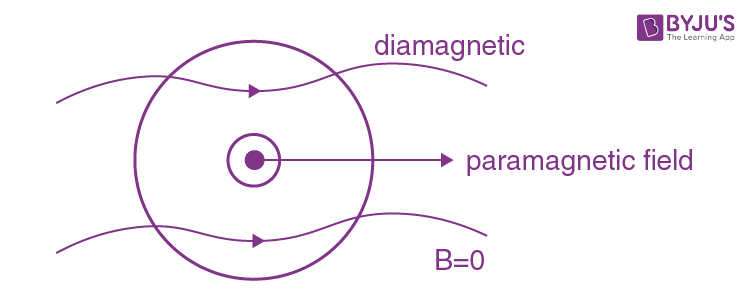15. Concentric metallic hollow spheres of radii R and 4R hold charges Q1 and Q2 respectively. Given that surface charge densities of the concentric spheres are equal, the potential difference V(R) – V(4R) is:

1)

$$\begin{array}{l}\frac{3Q_{2}}{4\pi \varepsilon _{0}R}\end{array}$$

2)
$$\begin{array}{l}\frac{3Q_{1}}{4\pi \varepsilon _{0}R}\end{array}$$

3)
$$\begin{array}{l}\frac{3Q_{1}}{16\pi \varepsilon _{0}R}\end{array}$$

4)
$$\begin{array}{l}\frac{Q_{1}}{4\pi \varepsilon _{0}R}\end{array}$$$$\begin{array}{l}\sigma =\frac{Q_{1}}{4\pi R^{2}}=\frac{Q_{2}}{4\pi 16R^{2}}\end{array}$$

16Q1=Q2

$$\begin{array}{l}V_{R}-V_{4R}=\frac{KQ_{1}}{R}+\frac{KQ_{2}}{4R}-\frac{KQ_{1}}{4R}-\frac{KQ_{2}}{4R}\end{array}$$
$$\begin{array}{l}=\frac{3KQ_{1}}{4R}=\frac{3Q_{1}}{16\pi \varepsilon _{0}R}\end{array}$$

16. The electric field of a plane electromagnetic wave propagating along the x direction in vacuum is

$$\begin{array}{l}\vec{E}=E_{0}\hat{j}cos(\omega t-kx)\end{array}$$
.The magnetic field
$$\begin{array}{l}\vec{B}\end{array}$$
, at the moment t = 0 is: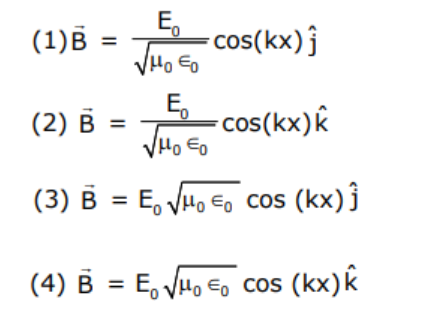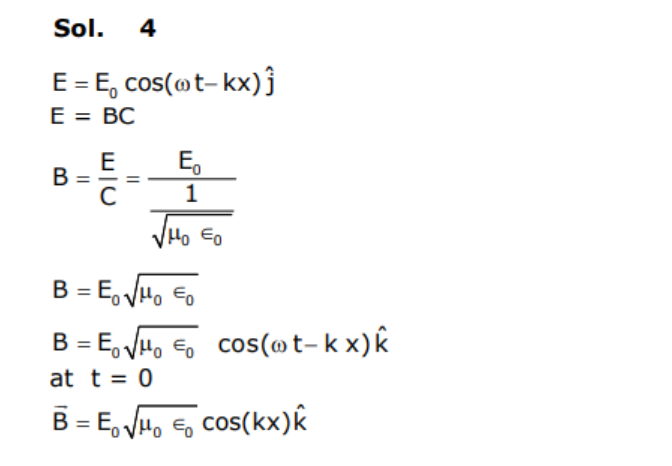17. A uniform magnetic field B exists in a direction perpendicular to the plane of a square loop made of a metal wire. The wire has a diameter of 4 mm and a total length of 30 cm. The magnetic field changes with time at a steady rate dB/dt = 0.032 Ts–1. The induced current in the loop is close to (Resistivity of the metal wire is 1.23 × 10–8 Ωm)

1) 0.53 A
2) 0.61 A
3) 0.34 A
4) 0.43 A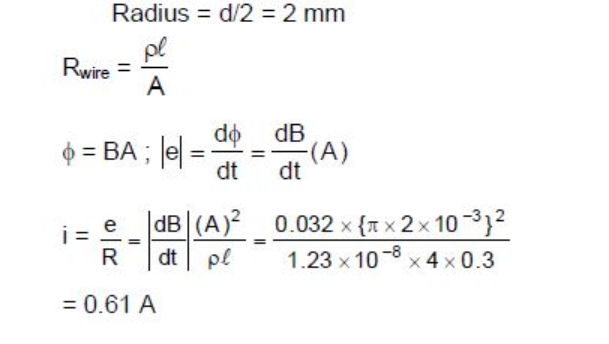18. Hydrogen ion and singly ionized helium atom are accelerated, from rest, through the same potential difference. The ratio of final speeds of hydrogen and helium ions is close to:

1) 2: 1
2) 1: 2
3) 5: 7
4) 10: 7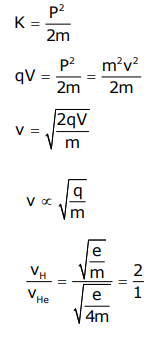19. Two light waves having the same wavelength λ in vacuum are in phase initially. Then the first wave travels a path L1 through a medium of refractive index n1 while the second wave travels a path of length L2 through a medium of refractive index n2. After this the phase difference between the two waves is:1)

$$\begin{array}{l}\frac{2\pi }{\lambda }(n_{1}L_{1}-n_{2}L_{2})\end{array}$$

2)
$$\begin{array}{l}\frac{2\pi }{\lambda }(\frac{L_{1}}{n_{1}}-\frac{L_{2}}{n_{2}})\end{array}$$

3)
$$\begin{array}{l}\frac{2\pi }{\lambda }(\frac{L_{2}}{n_{1}}-\frac{L_{1}}{n_{2}})\end{array}$$

4)
$$\begin{array}{l}\frac{2\pi }{\lambda }(n_{2}L_{1}-n_{1}L_{2})\end{array}$$

$$\begin{array}{l}\lambda _{n1}=\frac{\lambda }{n_{1}}\end{array}$$
$$\begin{array}{l}\lambda _{n2}=\frac{\lambda }{n_{2}}\end{array}$$
$$\begin{array}{l}(\Delta \phi )_{1}=\frac{2\pi}{\lambda _{n1}}L_{1}\end{array}$$
$$\begin{array}{l}(\Delta \phi )_{2}=\frac{2\pi}{\lambda _{n2}}L_{2}\end{array}$$
$$\begin{array}{l}\Delta \phi _{1}-\Delta \phi _{2}=\frac{2\pi}{\lambda }(n_{1}L_{1}-n_{2}L_{2})\end{array}$$

20. A calorimeter of water equivalent 20 g contains 180 g of water at 25°C. ‘m’ grams of steam at 100°C is mixed in it till the temperature of the mixture is 31°C. The value of ‘m’ is close to (Latent heat of water = 540 cal g–1, specific heat of water = 1 cal g–1 °C–1)

1) 2
2) 2.6
3) 4
4) 3.2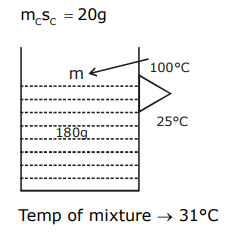Heat lost by steam = heat gained by water

180×1×(31-25)+20×(31-25)= m×540+m×1×(100-31)

180×6+20×6 = 540m + 100 m – 31m

1080+120 = 640 m – 31m

1200 = 609m

m = 1200/609

= 1.97

m = 2

21. If minimum possible work is done by a refrigerator in converting 100 grams of water at 0°C to ice, how much heat (in calories) is released to the surroundings at temperature 27°C (Latent heat of ice = 80 Cal/gram) to the nearest integer?

Q1= m L = 8000cal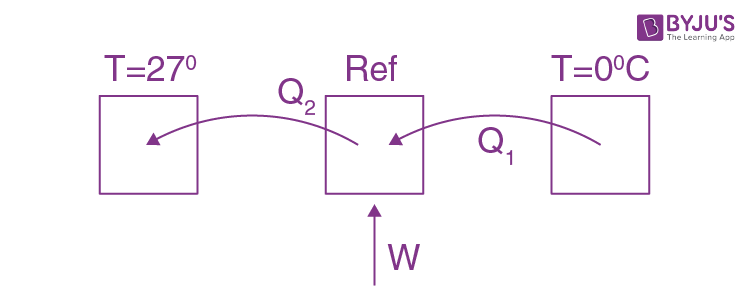Q2 = W + Q1

$$\begin{array}{l}C.O.P =\frac{Q_{1}}{W}=\frac{Q_{1}}{Q_{2}-Q_{1}}=\frac{T_{1}}{T_{2}-T_{1}}\end{array}$$
$$\begin{array}{l}\frac{Q_{1}}{W}=\frac{273}{27}\end{array}$$
$$\begin{array}{l}W = (27/273)Q_{1}\end{array}$$
$$\begin{array}{l}W = (27/273)\times 80 \times 100\end{array}$$
$$\begin{array}{l}Q_{2}=\frac{27}{273}\times 80\times 100+80\times 100\end{array}$$

= 8791.2 cal

22. An massless equilateral triangle EFG of side ‘a’ (As shown in figure) has three particles of mass m situated at its vertices. The moment of inertia of the system about the each line EX perpendicular to EG in the plane of EFG is (N/20) ma2 where N is an integer. The value of N is _____.Using parallel axis theorem

I = moment of inertia of triangle along the median + m(r2)

r=a/2

I = ma2+(ma2/4) = (5/4)ma2

(5/4)ma2= (N/20)ma2

N =25

23. A galvanometer coil has 500 turns and each turn has an average area of 3 × 10–4 m2. If a torque of 1.5 Nm is required to keep this coil parallel to a magnetic field when a current of 0.5 A is flowing through it, the strength of the field (in T) is ______.

$$\begin{array}{l}\tau =BINAsin\theta\end{array}$$

1.5 = B×0.5×500×3×10-4

B = (10000/500) = 20 Tesla

24. A block starts moving up an inclined plane of inclination 30° with an initial velocity of v0.It comes back to its initial position with velocity v0/2. . The value of the coefficient of kinetic friction between the block and the inclined plane is close to 1/1000 . The nearest integer to I is____.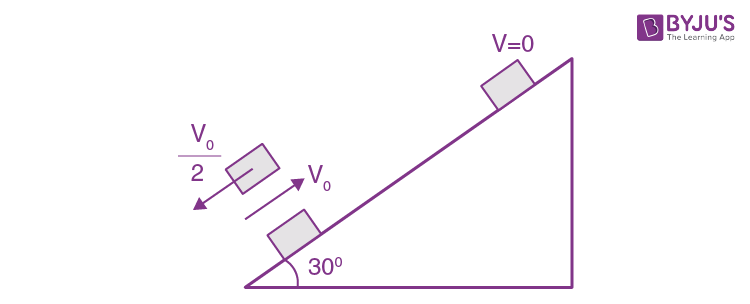a= gsin30+μ gcos 30(constant acceleration)

therefore, by applying kinematic formula

d= V02/2a

from work energy theorem

Wf = kf –ki

$$\begin{array}{l}-2\mu mgcos30\frac{V_{0}^{2}}{2a}=\frac{1}{2}m\frac{V_{0}^{2}}{4} – \frac{1}{2}mV_{0}^{2}\end{array}$$
$$\begin{array}{l}\frac{+2\mu mgcos30}{a}=+\frac{3}{4}\end{array}$$

8μgcos30 = 3gsin30+3 μgcos30

5μgcos30 = 3gsin30

$$\begin{array}{l}\mu =\frac{3tan30}{5}=\frac{\sqrt{3}}{5}\end{array}$$
$$\begin{array}{l}\frac{\sqrt{3}}{5}=\frac{I}{1000}\end{array}$$

I = 346

25. When an object is kept at a distance of 30 cm from a concave mirror, the image is formed at a distance of 10 cm from the mirror. If the object is moved with a speed of 9 cms–1, the speed (in cms–1) with which image moves at that instant is ____.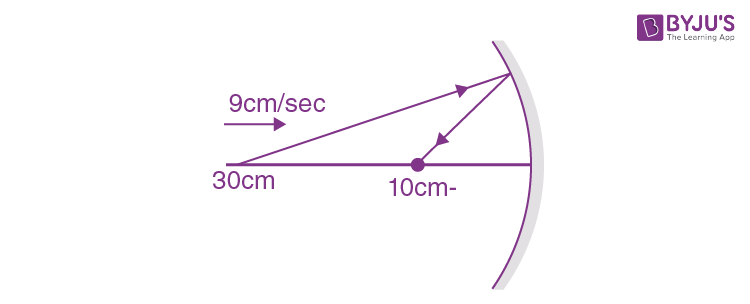$$\begin{array}{l}V_{1}=\frac{v^{2}}{u^{2}}v_{0}\end{array}$$
$$\begin{array}{l}V_{1}=\frac{10\times 10}{30\times 30}\times 9\end{array}$$